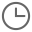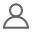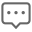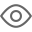400-990-8686

## PROTEL常用快捷键2016/03/18Greta010909

PageUp：以鼠标为中心放大

PageDown：以鼠标为中心缩小。

Home：将鼠标所指的位置居中

End：刷新（重画）

*：顶层与底层之间层的切换

+（-）逐层切换：“+”与“-”的方向相反

Qmm（毫米）与mil（密尔）的单位切换

IM：测量两点间的距离

Ex：编辑X，X为编辑目标，代号如下：(A)=圆弧；(C)=元件；(F)=填充；(P)=焊盘；(N)=网络；(S)=字符；(T)=导线；(V)=过孔；(I)=连接线；(G)=填充多边形。例如要编辑元件时按EC，鼠标指针出现“十”字，单击要编辑的元件即可进行编辑。

Px：放置X，X为放置目标，代号同上。

Mx：移动X，X为移动目标，(A)、(C)、(F)、(P)、(S)、(T)、(V)、(G)同上，另外(I)=翻转选择部份；(O)旋转选择部份；(M)=移动选择部份；(R)=重新布线。

Sx：选择X，X为选择的内容，代号如下：(I)=内部区域；(O)=外部区域；(A)=全部；(L)=层上全部；(K)=锁定部分；(N)=物理网络；(C)=物理连接线；(H)=指定孔径的焊盘；(G)=网格外的焊盘。例如要选择全部时按SA，所有图形发亮表示已被选中，可对选中的文件进行复制、清除、移动等操作。400-990-8686